Home MathLab Scalar Functions in Matlab: E Matlab, Cosine Function and More

# Scalar Functions in Matlab: E Matlab, Cosine Function and More

These are a few basic tools you will need to know if you are just starting learning Matlab.

## Scalar functions in Matlab

Before fully diving into trigonometric functions, it is necessary to state that Matlab takes as input radians while working with trigonometric function meaning and uses the symbol pi for π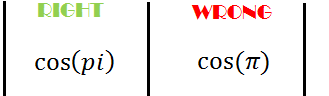### Trigonometric sine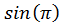Matlab code

`sin(pi)`

### Trigonometric cosine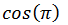Matlab code

`cos(pi)`

###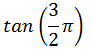Matlab code

`tan(3*pi/2)`

###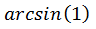Matlab code

`asin(1)`

###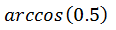Matlab code

`acos(0.5)`

###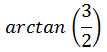Matlab code

`atan(3/2)`

Matlab code

`exp(x)`

###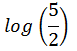Matlab code

`log(5/2)`

###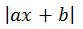Matlab code

`abs(a*x+b)`

###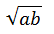Matlab code

`sqrt(a*b)`

### Remainder after division

This function helps you find the remainder after a division. We will use as an example the remainder of the division of 5 by 2, which is one.

Matlab code

`rem(5,2)`

which returns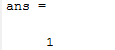### Round towards nearest integer

This function will allow you to round values towards the nearest integer if you are not interested in working with decimal numbers. It is most useful while you want to round the result of an operation.

Matlab code

`round(2.9)`

Which returns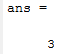### Round towards negative infinity

floor(x) rounds the elements of x to the nearest integers towards minus infinity.

### Round towards positive infinity

As opposed to the floor function, ceil(x) rounds the elements of x to the nearest integers towards infinity.

### Articles you might like:

#### Related Posts

This website uses cookies to improve your experience. We'll assume you're ok with this, but you can opt-out if you wish. Accept Read More